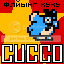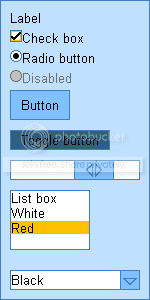-=+=- -=+=- -=+=- -=+=- -=+=- -=+=- -=+=- -=+=- -=+=- -=+=- -=+=- -=+=- -=+=- -=+=- -=+=- -=+=- -=+=- -=+=- -=+=- -=+=- -=+=- -=+=- -=+=- -=+=- -=+=- -=+=- -=+=- -=+=- -=+=- -=+=- (c) WidthPadding Industries 1987 0|60|0 -=+=- -=+=- -=+=- -=+=- -=+=- -=+=- -=+=- -=+=- -=+=- -=+=- -=+=- -=+=- -=+=- -=+=- -=+=- -=+=- -=+=- -=+=- -=+=- -=+=- -=+=- -=+=- -=+=- -=+=- -=+=- -=+=- -=+=- -=+=- -=+=- -=+=-### flying_cucco

View Profile, Send Private Message
Recent Posts, Showcases, Articles, Code Snippets, Links, Wed.Workshop, Blog

### Latest Blog Entries

flying_cucco# Homeopathy

22nd February 2010(Image.PNG)

### Latest Showcases

flying_cucco# Dress-up

16th September 2008For WW #168

flying_cucco# Opalescence

29th June 2008A small GUI toolkit for Cobra

flying_cucco# Cuccos Tools

18th May 2008

Webserver, database and crawler for IK tools site.

flying_cucco# MapEdit

12th May 2007

Abandoned tilemap editor